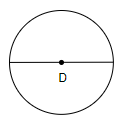Courses
Courses for Kids
Free study material
Offline Centres
MoreLast updated date: 01st Dec 2023
Total views: 357.9k
Views today: 9.57k

# A bicycle wheel makes 5,000 revolutions in moving 11km. Find the diameter of the wheel. (Take $\pi =\dfrac{22}{7}$ )Verified
357.9k+ views
Hint: We will first find the distance covered by the wheel in one revolution, that is the circumference of the wheel, by using the formula, $\dfrac{\text{distance covered}}{\text{total no}\text{. of revolution}}$. We know that the circumference of a circle is equal to $2\pi r$, where r is the radius of the circle. From the equation, we will get the circumference of the wheel, hence on equating it, we will get the radius of the wheel, then we will get the diameter of the wheel as 2 (radius).

It is given in the question that a bicycle wheel makes 5,000 revolutions in moving 11km. And we have been asked to find the diameter of the wheel.
Now, let us consider the figure of a wheel with the diameter D.We are given that the total distance covered by the wheel is 11km and the total number of revolutions is 5,000. We will convert these values into cm for calculations.
So, we know that, 1 km = 100000 cm. So, $11km=11\times 100000=1100000cm$.
Now, we know that in 1 revolution, the distance covered by the wheel can be found out as, $\dfrac{\text{distance covered}}{\text{total no}\text{. of revolution}}$.
On substituting the values of distance covered as 1100000 cm and the no. of revolutions as 5,000 in the above equation, we get,
$\dfrac{1100000}{5000}=220cm$
Thus, the wheel will cover 220cm in one complete revolution. That is the circumference of the wheel is 220cm.
We know that the circumference of a circle is given by the formula, $2\pi r$, where r is the radius of the circle. So, we can write the circumference of the wheel as, $2\pi r$.
We also have found out the value of the circumference of the wheel as 220 cm. So, we can write,
$2\pi r=220$
On dividing both the sides by $2\pi$, we will get,
$r=\dfrac{220}{2\pi }$
On substituting the value of $\pi =\dfrac{22}{7}$, we get,
\begin{align} & r=\dfrac{220}{2\times \dfrac{22}{7}} \\ & r=\dfrac{220\times 7}{2\times 22} \\ & r=35cm \\ \end{align}
So, we get the radius of the wheel as 35cm.
Now, we know that dimeter = 2 (radius). So, we get the diameter of the wheel as $2\times 35cm=70cm$.
Therefore, the diameter of the bicycle wheel is 70cm.

Note: While solving this question, most of the students get confused between the formula of circumference and area of a circle and as a result, they often take $\pi {{r}^{2}}$ as the circumference of the circle and will end up getting the wrong answer. So, the students must remember that $\pi {{r}^{2}}$ is the formula of the area of a circle and $2\pi r$ is the formula of the circumference of a circle. Also, the students must understand that, in 1 revolution of a wheel, the distance covered by the wheel will be equal to the circumference of the wheel itself. Also, when it is given in the question that we have to take $\pi =\dfrac{22}{7}$, then do so, or else there will be slight variations in the final answer.